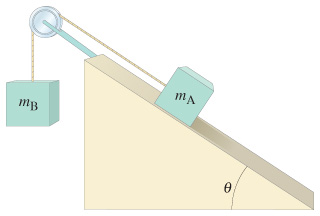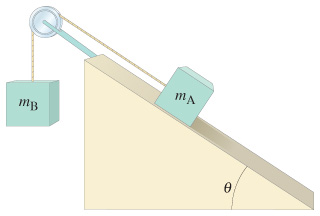# Problem: Suppose the coefficient of kinetic friction between mA and the plane in the figure is μk = 0.13, and that mA = mB = 2.7 kg.(a) As mB moves down, determine the magnitude of the acceleration of mA and mB, given  θ = 33°.(b) What value of μk will keep the system from accelerating?

###### FREE Expert Solution

This problem asks us to determine the magnitude of acceleration of the system for a given coefficient of kinetic friction, then the smallest value of μk for which the system has zero acceleration.

This is a system of objects, inclined planes with friction kind of problem. Just as forces problems, we'll follow the same series of steps:

1. Draw free body diagrams (FBDs) for the objects of interest
2. Apply Newton's 2nd Law (Fma )
3. Solve for the target variable

The kinetic friction force is calculated using

$\overline{){{\mathbit{f}}}_{{\mathbit{k}}}{\mathbf{=}}{{\mathbit{\mu }}}_{{\mathbit{k}}}{\mathbit{N}}}$

Also, remember that when we use tilted coordinate axes for inclined plane problems like this, the weight mg makes a known angle with the y-axis, not the x-axis. That means we'll decompose block A's weight vector like this:

95% (53 ratings)###### Problem Details

Suppose the coefficient of kinetic friction between mA and the plane in the figure is μk = 0.13, and that mA = mB = 2.7 kg.

(a) As mB moves down, determine the magnitude of the acceleration of mA and mB, given  θ = 33°.

(b) What value of μk will keep the system from accelerating?